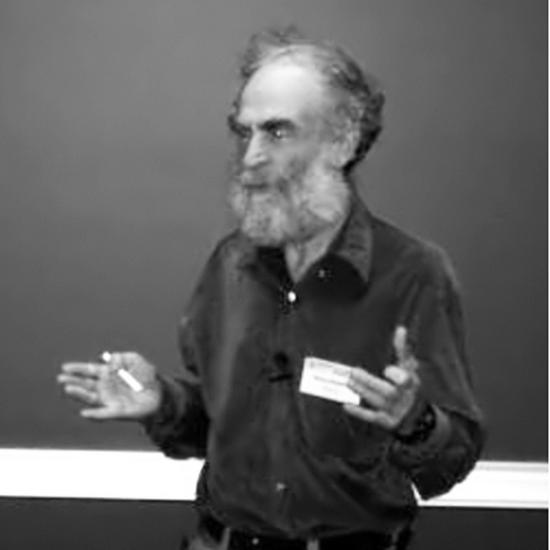# Mikhail GromovForeign Member

Elected: 2011

## Biography

Mikhail Gromov is a mathematician who has profoundly influenced many fields, including geometry, differential equations and probability theory. In particular, he is considered to have revolutionised Riemannian geometry, which has important applications in branches of physics such as general relativity. Mikhail is also credited with spurring the establishment of geometric group theory as a distinct area of mathematics.

His research is marked by deep insights as to how questions in one mathematical area can be tackled using techniques from another. He is particularly noted for doing so in geometrical terms. Recently, he has turned his attention to biology, after noting a similarity between mathematical objects called hyperbolic Markov partitions and the process of cell division.

Mikhail is the recipient of many distinguished awards, including: the 1993 Wolf Prize in Mathematics; the 1999 Balzan Prize for Mathematics; the 2004 Frederic Esser Nemmers Prize in Mathematics; and the 2002 Kyoto Prize for Basic Sciences. In 2009, he won the Abel Prize for his “revolutionary contributions to geometry”.

## Awards

• #### Balzan Prize

For mathematics.

• #### Kyoto Prize

In the field of mathematical sciences.

• #### Wolf Prize

In the field of mathematics for his revolutionary contributions to global Riemannian and symplectic geometry, algebraic topology, geometric group theory and the theory of partial differential equations.